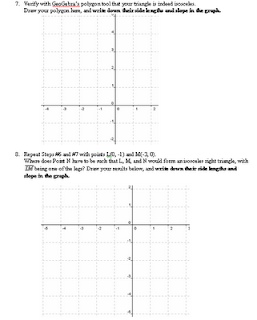## Tuesday, October 19, 2010

### Tilted Parallelogram, Triangles, and Good Ol' Slope

Last year, when I taught parallel and perpendicular lines in Geometry, I took the kids to the computer lab and did some investigation via GeoGebra so that they can observe for themselves that the perpendicular lines indeed have reciprocal slopes, etc. This year, I'll probably do that again with the regular Geometry kids, but with the honors kids (who can already recall from last year how to write parallel and perpendicular slopes), I am skipping over that and giving them a more challenging GeoGebra investigation right away. This new task will ask them to complete parallelograms and tilted right triangles in the coordinate plane, thereby making them apply their knowledge of parallel and perpendicular slopes in order to find the missing vertices. (ie. If you are given three vertices in a parallelogram, where does the fourth vertex have to be? Are there multiple possibilities?) They can use a simple built in polygon tool in GeoGebra to verify that certain sides in their parallelograms (and/or in their isosceles right triangles) are indeed congruent.

Example problems from the activity (slightly rephrased):
• If A(1, 1), B(2, -2), C(4, 3) are three vertices inside a parallelogram, where is the fourth vertex, D? (Are there multiple possibilities?)

• If I(-1, 3) and J(0, 1) are two vertices in an isosceles right triangle, where is the third vertex, K? (Are there multiple possibilities?)

• Do the vertices M(-2, 5), J(0, 1), and K(the answer to the previous problem) make an acute, obtuse, or right triangle?

• Do the vertices N(-1, 4), J(0, 1), and K(as before) make an acute, obtuse, or right triangle?

I will also give the kids some already-drawn triangles at the end of the investigation, for them to find the slopes in order to determine whether those are acute, obtuse, or actually right triangles. (Because on my last test, some kids drew some triangles that looked sort of like right triangles but were actually not, when you take a closer look at their slopes. I took only a couple of points off then for their "right" triangles, since we hadn't explicitly talked about right triangles in connection with the slopes of their edges. In the future, the kids should be able to catch those mistakes on their own.)

I am excited about this, because it is asking kids to geometrically apply/extend their understanding of slopes, and it also paves the way to coordinate proofs, which are just around the corner.